# 结对编程作业

## Github项目地址

https://github.com/lwnlwn123/lwn1

## 具体分工

031802610 赖彪 博客链接 Github 原型设计80%，AI实现20%
031802621 刘惟凝 博客链接 https://github.com/lwnlwn123/lwn1 原型设计20%，AI实现80%

# 1.1 - 原型设计

## [1.1.1]此次结对作业的设计说明：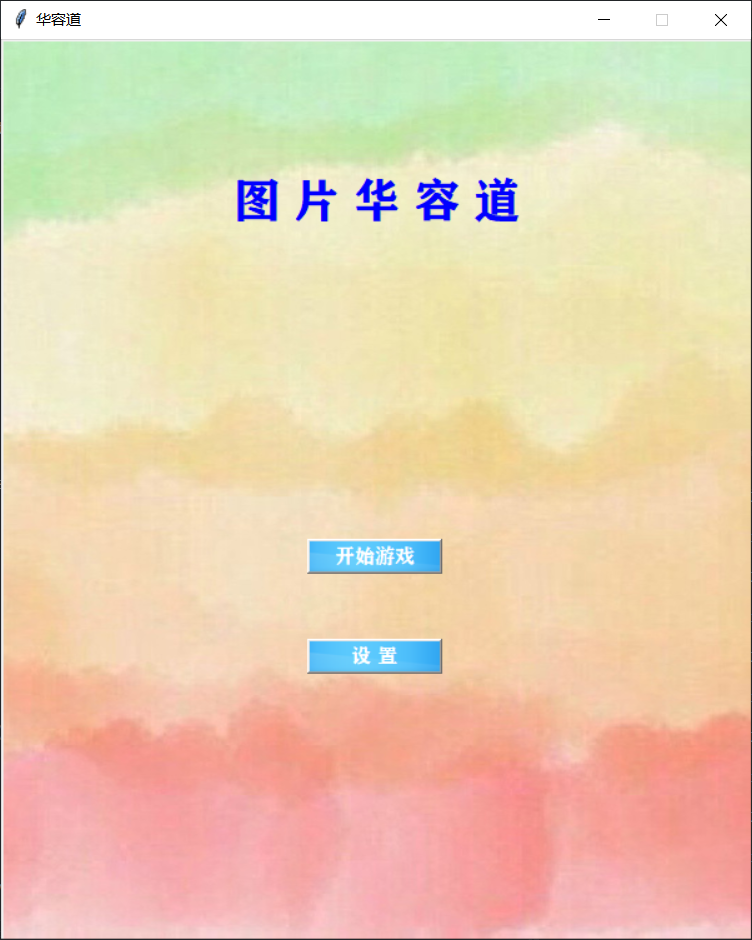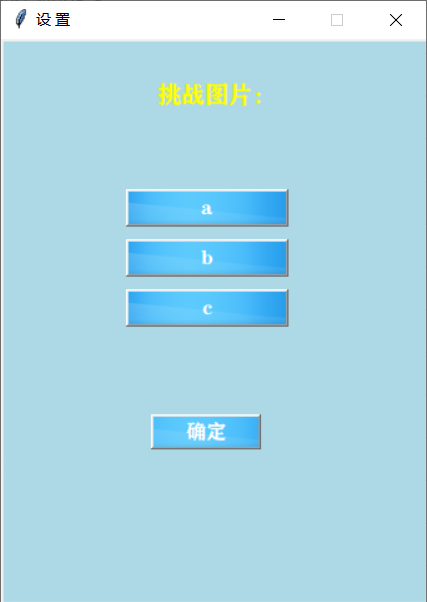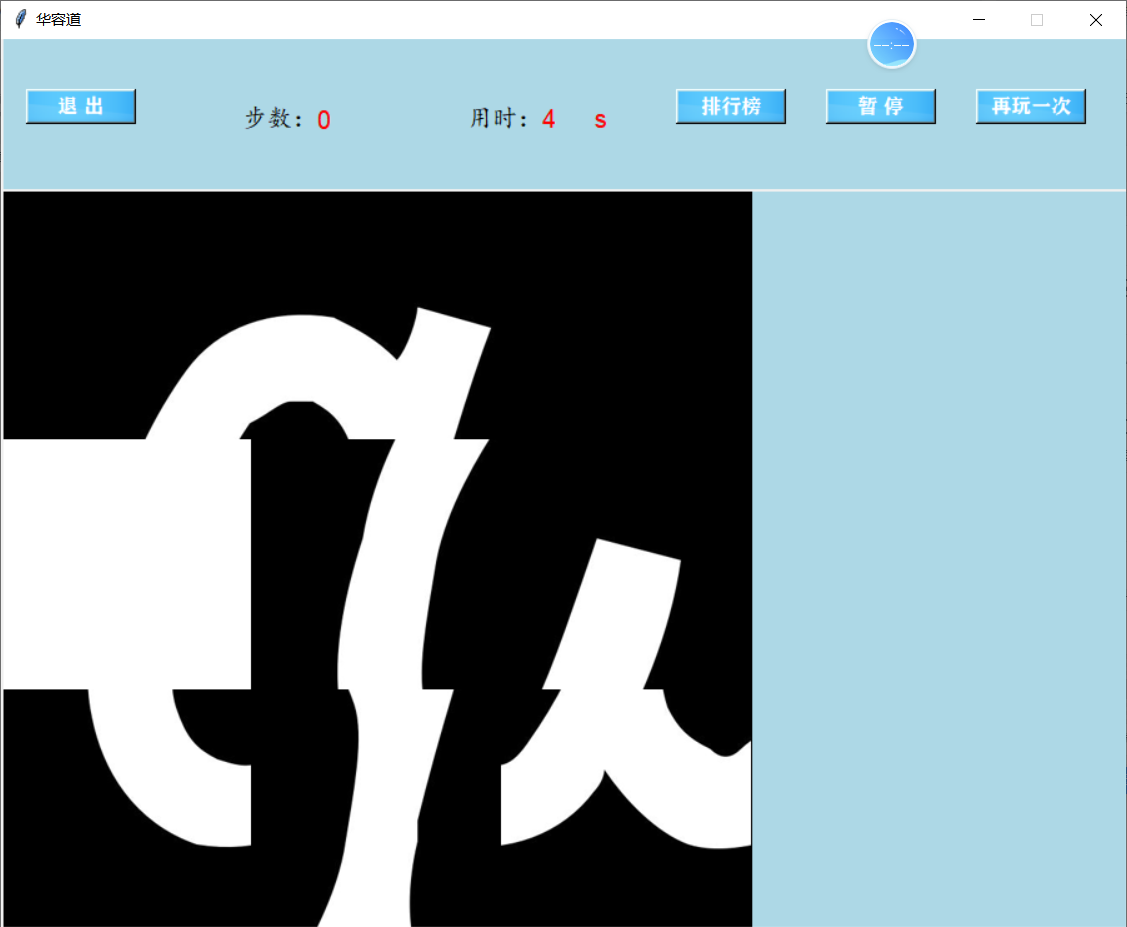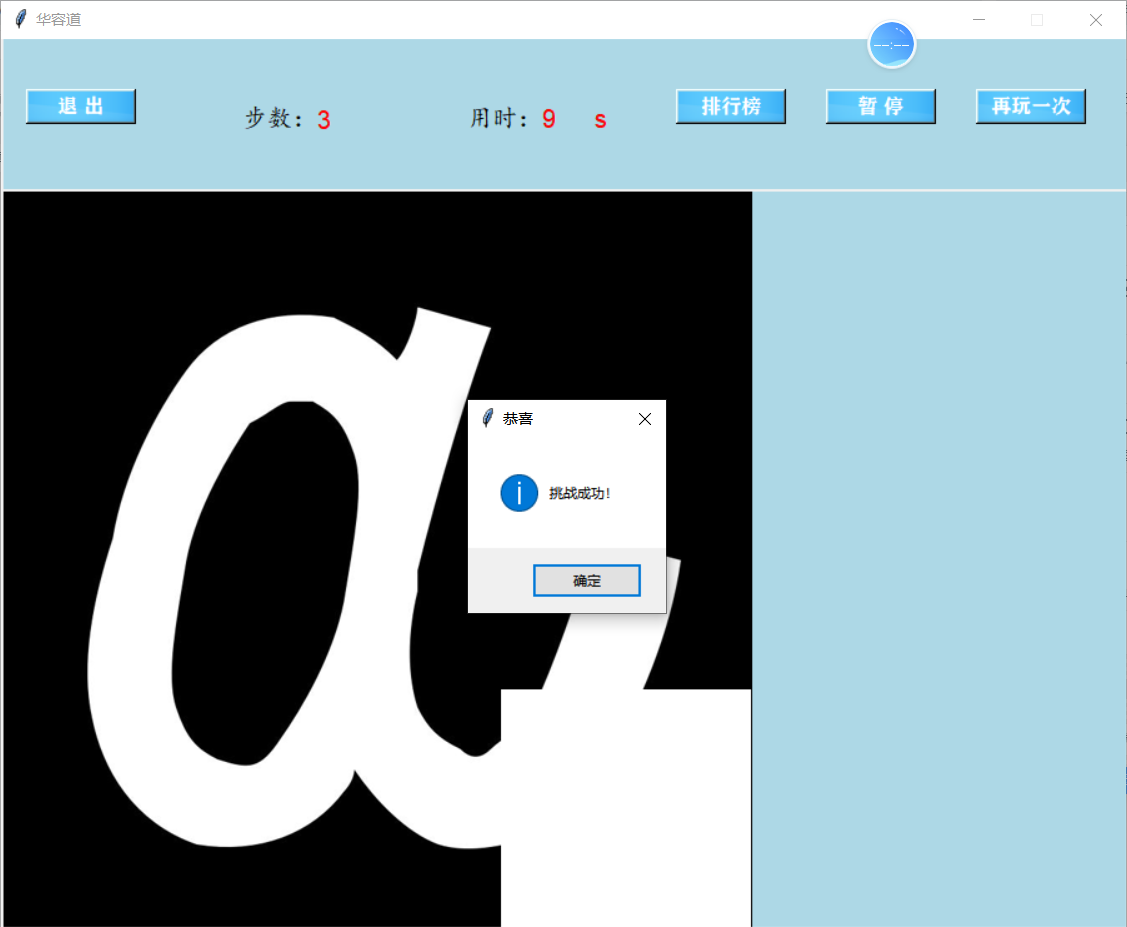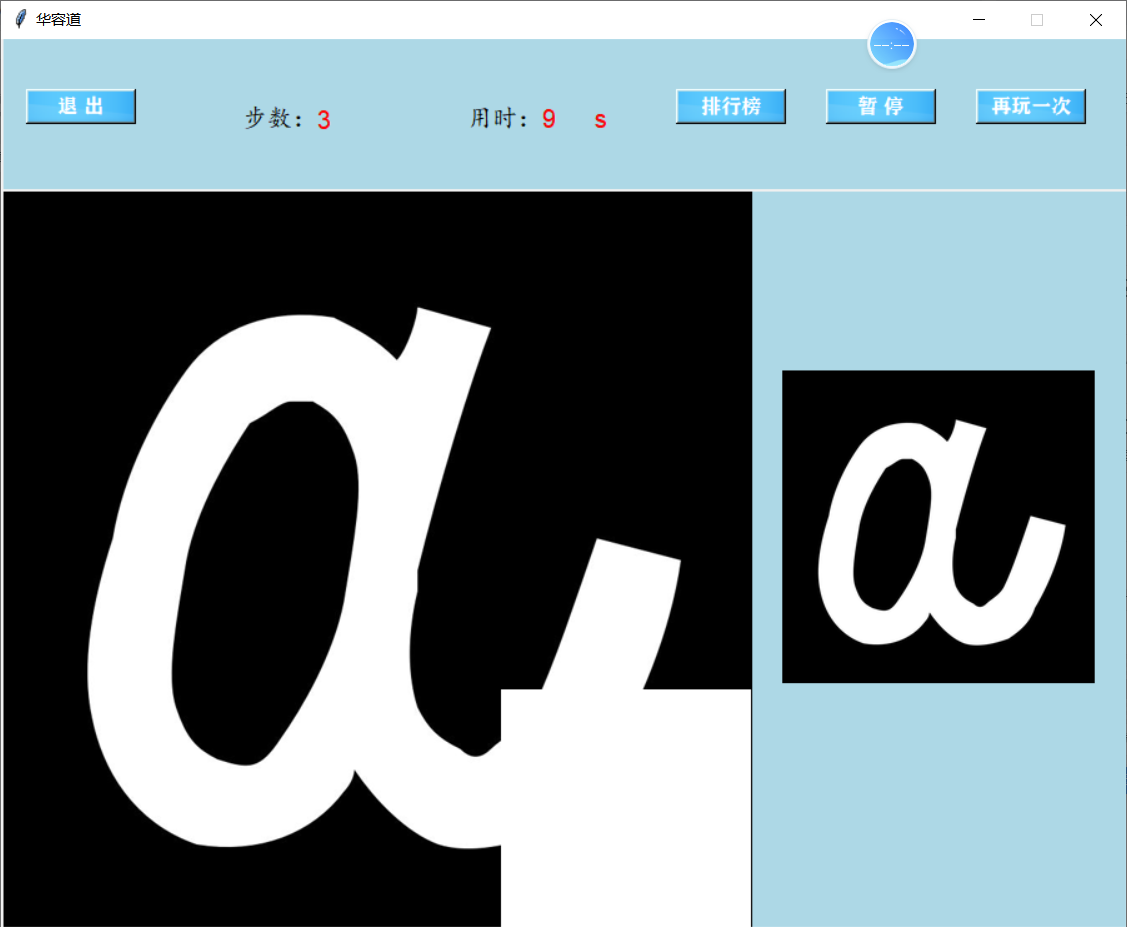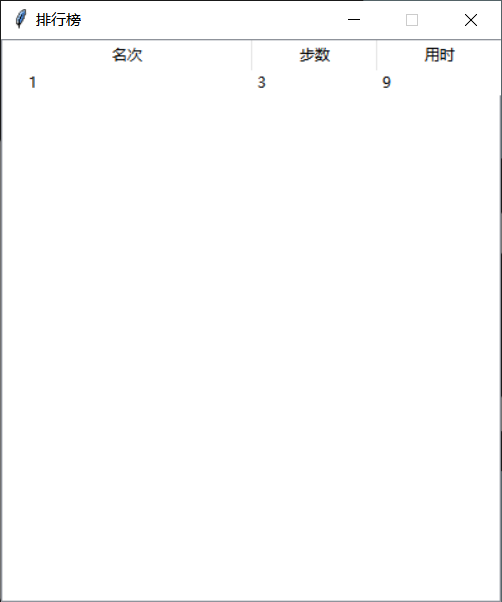Spyder

## [1.1.3]描述结对的过程：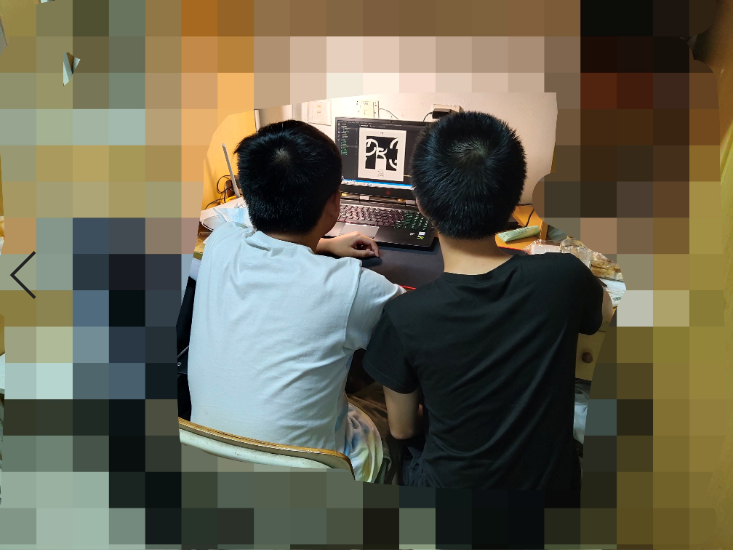## [1.2.1]代码实现思路：

python的requests库

#图片匹配函数，返回的result值越小，图片越相似，若resul=0，则两张图完全一样
from PIL import Image
import math
import operator
from functools import reduce

def image_contrast(img1,img2):

image1=Image.open(img1)
image2=Image.open(img2)

h1=image1.histogram()
h2=image2.histogram()

return result

#通过键盘控制图片移动的函数，sq用于图片的绘制，sqqq用于胜利的判定
def keyboard(event):
global step,cv,mark_col,mark_row,operates
if event.keysym=="w" or event.keysym=="Up":
if mark_row!=0:
temp=sq[mark_row-1][mark_col]
sq[mark_row-1][mark_col]=sq[mark_row][mark_col]
sq[mark_row][mark_col]=temp
temp2=sqqq[3*(mark_row-1)+mark_col]
sqqq[3*(mark_row-1)+mark_col]=sqqq[3*(mark_row)+mark_col]
sqqq[3*(mark_row)+mark_col]=temp2
mark_row-=1
operates+="w"
step+=1
print(sqqq)
print(operates)
elif event.keysym=="a" or event.keysym=="Left":
if mark_col!=0:
temp=sq[mark_row][mark_col-1]
sq[mark_row][mark_col-1]=sq[mark_row][mark_col]
sq[mark_row][mark_col]=temp
temp2=sqqq[3*(mark_row)+mark_col-1]
sqqq[3*(mark_row)+mark_col-1]=sqqq[3*(mark_row)+mark_col]
sqqq[3*(mark_row)+mark_col]=temp2
mark_col-=1
operates+="a"
step+=1
print(sqqq)
print(operates)
elif event.keysym=="s" or event.keysym=="Down":
if mark_row!=2:
temp=sq[mark_row+1][mark_col]
sq[mark_row+1][mark_col]=sq[mark_row][mark_col]
sq[mark_row][mark_col]=temp
temp2=sqqq[3*(mark_row+1)+mark_col]
sqqq[3*(mark_row+1)+mark_col]=sqqq[3*(mark_row)+mark_col]
sqqq[3*(mark_row)+mark_col]=temp2
mark_row+=1
operates+="s"
step+=1
print(sqqq)
print(operates)
elif event.keysym=="d" or event.keysym=="Right":
if mark_col!=2:
temp=sq[mark_row][mark_col+1]
sq[mark_row][mark_col+1]=sq[mark_row][mark_col]
sq[mark_row][mark_col]=temp
temp2=sqqq[3*(mark_row)+mark_col+1]
sqqq[3*(mark_row)+mark_col+1]=sqqq[3*(mark_row)+mark_col]
sqqq[3*(mark_row)+mark_col]=temp2
mark_col+=1
operates+="d"
step+=1
print(sqqq)
print(operates)
label_step["text"]=str(step)
cv.delete('all')
draw_image(cv)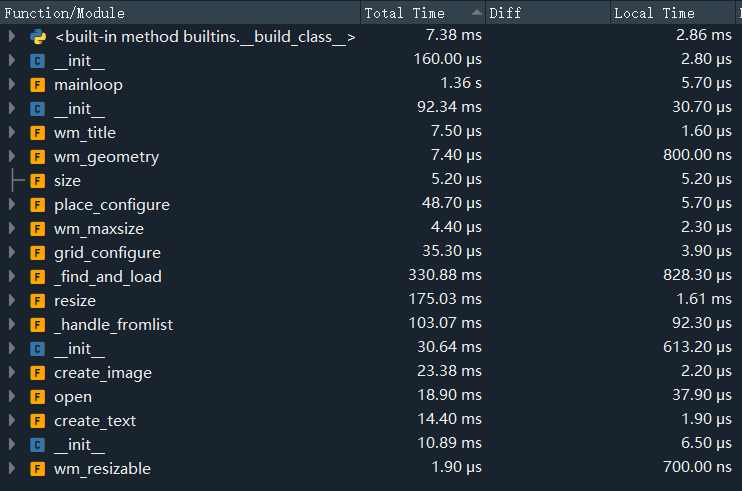AI: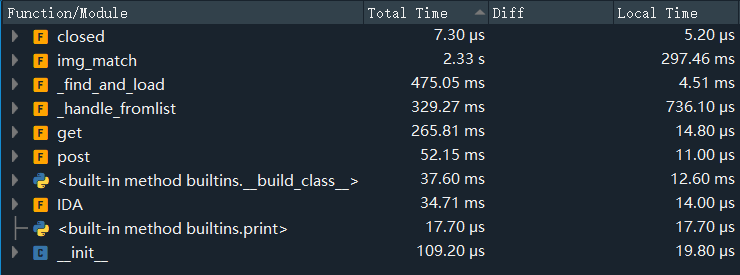none

## [1.2.5]提供此次结对作业的PSP和学习进度条（每周追加）

PSP2.1 Personal Software
Process Stages

（分钟）

（分钟）
Planning 计划
· Estimate · 估计这个任务需要多少

30 30
Development 开发
· Analysis · 需求分析 (包括学习新技

900 1380
· Design Spec · 生成设计文档 20 40
· Design Review · 设计复审 20 30
· Coding Standard · 代码规范 (为目前的开发

20 30
· Design · 具体设计 200 230
· Coding · 具体编码 600 750
· Code Review · 代码复审 60 50
· Test · 测试（自我测试，修改

100 120
Reporting 报告
· Test Repor · 测试报告 20 20
· Size Measurement · 计算工作量 20 15
· Postmortem & Process
Improvement Plan
· 事后总结, 并提出过程改

20 30
Total 合计 1980 2725

（行）

（行）

（小时）

（小时）

1 68 68 7 7 Python、Pillow
2 89 157 5 12 Request、GUI
3 59 216 5 17 -
4 72 288 6 23 BFS相关
posted @ 2020-10-19 22:47  lwn_user  阅读(130)  评论(0编辑  收藏  举报
levels of contents